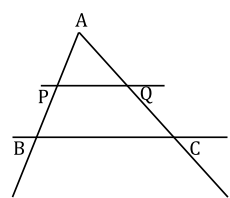# Free SAT Test Questions

Question 1 of 1
ID: SAT-AM-3
Section: Math - Additional Topics in Math
Topic: Geometry
Difficulty level: Challenging

(Practice Mode: Single selected Question » Back to Overview)

In the given graphic $\Delta ABC$, $PQ{\parallel}BC$ and AP : PB = 3 : 2. If the area of $\Delta APQ$ is 27 sq. units, what is the area of $\Delta ABC$ (in sq. units)? (With calculator)